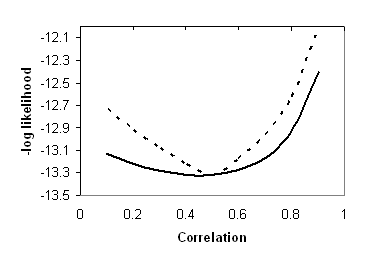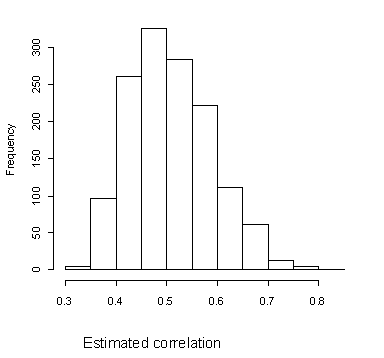## Sunday, 28 August 2011

### Estimating standard errors

Estimation of standard errors of paths is a highly technical, and sometimes contentious topic. Here we attempt to give some insight into this topic without going into mathematical details. One way of determining a standard error is to inspect the likelihood function. Think back to our earlier Likelihood_demo script, which estimated the likelihood of a single correlation. Figure 5 shows two likelihood functions: the unbroken line shows a shallow function, whereas the dotted line shows a more peaked function. Typically, the larger the sample, the more peaked the function will be. For the shallower function, the confidence interval around the estimate of the correlation will be broader than for the more peaked function. The 'gradient' of the likelihood function is thus a key factor in determining standard errors. You may see reference to the term "Hessian matrix" in OpenMx output: this is a matrix that is used in estimating the likelihood gradient.Figure 5: Likelihood functions with steep and shallow gradients

An alternative method for estimating standard errors is by bootstrapping. This involves repeatedly simulating data with a true value of a parameter (e.g. a correlation of .5) and then looking at the range of estimates that are obtained by model-fitting. For instance, if we simulate 10,000 datasets and run each of them through our model, we won't get the same parameter estimate every time. The distribution of estimates may look something like this:Figure 6: Estimates of correlation from repeated simulations with r = .5
We can then get a precise value for confidence limits: for instance, if we find the bottom 2.5% of estimates and the top 2.5% of estimates; the 95% CI will be the range between these limits. Note that when estimated this way, the confidence interval need not be symmetric around the estimated value. Bootstrapping is growing in popularity as a method for assessing confidence intervals, because it directly estimates the probability of different values of a statistic in repeated model runs, and does not depend on any assumptions about distributions of statistics. However, for complex models where model optimization takes more than a few seconds, it can become unfeasibly slow. For instance, if it takes two minutes to run a model, then to re-run it 10,000 times to get repeated estimates of the parameters would take nearly two weeks.